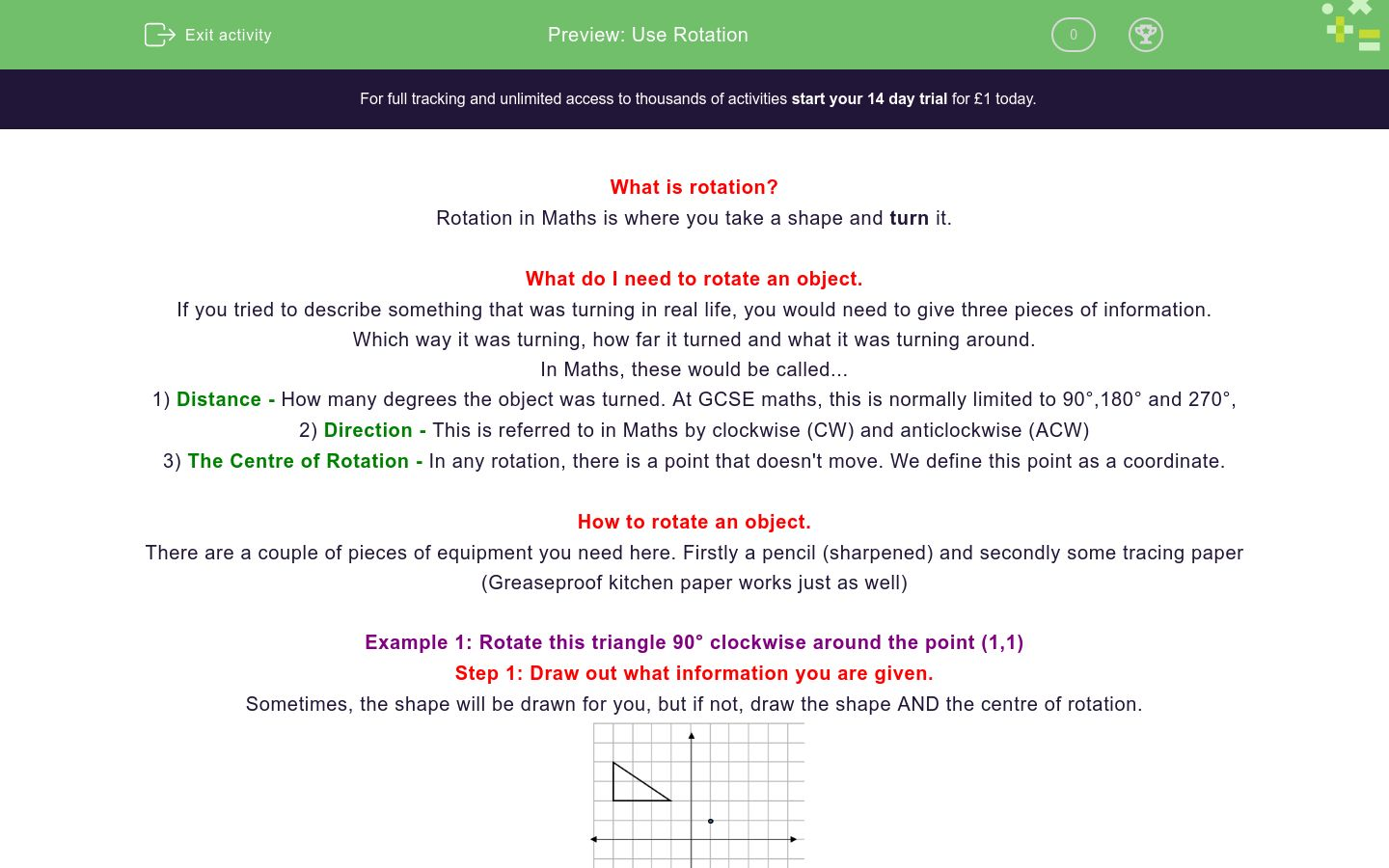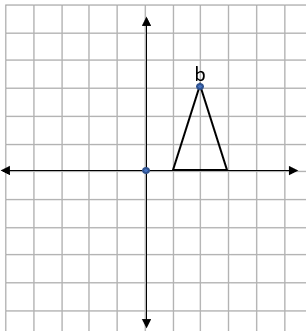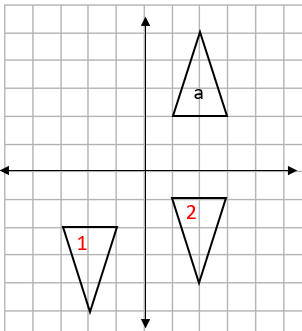# Use Rotation

In this worksheet, students practise rotating an object.Key stage:  KS 4

GCSE Subjects:   Maths

GCSE Boards:   AQA, Eduqas, Pearson Edexcel, OCR

Curriculum topic:   Geometry and Measures, Congruence and Similarity

Curriculum subtopic:   Properties and Constructions, Plane Vector Geometry

Difficulty level:### QUESTION 1 of 10

What is rotation?

Rotation in Maths is where you take a shape and turn it.

What do I need to rotate an object?

If you tried to describe something that was turning in real life, you would need to give three pieces of information.

Which way it was turning, how far it turned and what it was turning around.

In Maths, these would be called...

1) Distance - How many degrees the object was turned. At GCSE maths, this is normally limited to 90°,180° and 270°,

2) Direction - This is referred to in Maths by clockwise (CW) and anticlockwise (ACW)

3) The Centre of Rotation - In any rotation, there is a point that doesn't move. We define this point as a coordinate.

How to rotate an object.

There are a couple of pieces of equipment you need here. Firstly a pencil (sharpened) and secondly some tracing paper (Greaseproof kitchen paper works just as well)

Example 1: Rotate this triangle 90° clockwise around the point (1,1)

Step 1: Draw out what information you are given

Sometimes, the shape will be drawn for you, but if not, draw the shape AND the centre of rotation.Step 2: Trace

Put your tracing paper over your shape, making sure you cover the centre of rotation.

I would recommend that when you trace the centre, draw a cross over the centre, this makes it easy to see if you have rotated exactly 90°.Step 3: Complete the rotation

Place the point of your pencil on the centre of rotation (this makes sure it doesn't move while you turn the tracing paper) and turn the tracing paper 90° clockwise.Step 4: Transfer the image and label

The easiest way to transfer the shape back onto the paper is to press on the corners of the shape with your pencil (do this lightly)

This will give three little dips in the paper underneath that you can just join up to finish the shape.

Remember that if the image is labeled a, the rotation will be labeled a'When we are rotating an object, we normally need three pieces of information...

Rotation of 180° does not require direction because a shape would end up in  ........ position.

the same

a different

the opposite

Rotating 90° clockwise is the same as rotating...

180 °

240 °

270 °

260 °

This shape has been rotated anticlockwise. How far has it been rotated?(Don't put in the degree sign, just concentrate on getting the number correct)

180 °

240 °

270 °

260 °

Which of the transformations below could describe this transformation?90° clockwise

90° anti-clockwise

180°

270° clockwise

270° anti-clockwise

Is this a clockwise or an anticlockwise rotation?Clockwise

Anticlockwise

We can't tell

I rotate this shape 90° clockwise around the origin.

What are the new co-ordinates of b'?HIN: Try drawing it out first.

Clockwise

Anticlockwise

We can't tell

I rotate this shape 180° around the origin.

What are the co-ordinates of b'?HIN: Try drawing it out first.

Clockwise

Anticlockwise

We can't tell

I rotate shape a 180° around the point (2,1)

Which of the two rotations is correct?Shape 1

Shape 2

Neither

Shape a is rotated 180° around the point (1,-1)

Which of the two rotations is correct?Shape 1

Shape 2

• Question 1

When we are rotating an object, we normally need three pieces of information...

EDDIE SAYS
How did you get on? You need to remember these three definitions if you want to get really good at using rotation in your work! When you come to describing a rotation, you need to give all three to get full marks.
• Question 2

Rotation of 180° does not require direction because a shape would end up in  ........ position.

the same
EDDIE SAYS
Try it. Take something (a book, a pen etc), turn it 180 clockwise and then 180 anticlockwise. It'll end up in the same position!
• Question 3

Rotating 90° clockwise is the same as rotating...

270 °
EDDIE SAYS
Turning 1/4 turn one way is exactly the same as turning 3/4 of a turn the other way. If 90 ° is a quarter, then 270 ° = three quarters. As before, if you want to test it out, give it a go!
• Question 4

This shape has been rotated anticlockwise. How far has it been rotated?(Don't put in the degree sign, just concentrate on getting the number correct)

EDDIE SAYS
Did you read the question carefully? If we are going anticlockwise, the shape has been turned 3/4 of a full turn. How many degrees is this the same as?
• Question 5

Which of the transformations below could describe this transformation?90° clockwise
270° anti-clockwise
EDDIE SAYS
Have you spotted the pattern? Whenever you do a rotation, there are always two ways to describe this, as you can move in two directions. For this one, I could have done 1/4 clockwise or 3/4 anticlockwise
• Question 6

Is this a clockwise or an anticlockwise rotation?We can't tell
EDDIE SAYS
Did you get this one right? The question asks you if you can see which direction the rotation has taken place in. It could have been anti-clockwise or clockwise. There are always two ways you could have done the rotation. BUT, without more information, we can't say which way it has turned.
• Question 7

I rotate this shape 90° clockwise around the origin.

What are the new co-ordinates of b'?HIN: Try drawing it out first.

EDDIE SAYS
A couple of things to remember here. 1) The origin is the point (0,0) 2) b' is the rotated version of point b If you are having a tough time seeing this, why not draw it out and try turning your page?
• Question 8

I rotate this shape 180° around the origin.

What are the co-ordinates of b'?HIN: Try drawing it out first.

EDDIE SAYS
How did you get on? Did you visualise it or try drawing it out? A couple of things to remember here. 1) The origin is the point (0,0) 2) b' is the rotated version of point b
• Question 9

I rotate shape a 180° around the point (2,1)

Which of the two rotations is correct?Neither
EDDIE SAYS
Did you get caught out here? The easiest way to do this is to draw it out and do it yourself. The mistake often made here is to rotate around the origin instead of the point that is given. (this is where shape 1 has come from). Shape 2 is also incorrect as if it is rotated correctly around the coordinate (2,1) it should sit along the x axis.
• Question 10

Shape a is rotated 180° around the point (1,-1)

Which of the two rotations is correct?Shape 1
EDDIE SAYS
How are you feeling about rotation now? The easiest way to do this is to draw it out and do it yourself. Be very careful when plotting your centre. Shape 1 has been rotated around (1, -1) whereas, shape 2 has been rotated about (-1, 1) Can you see how much of a difference plotting your coordinates the wrong way round can make? That's another activity ticked off! Excellent progress.
---- OR ----